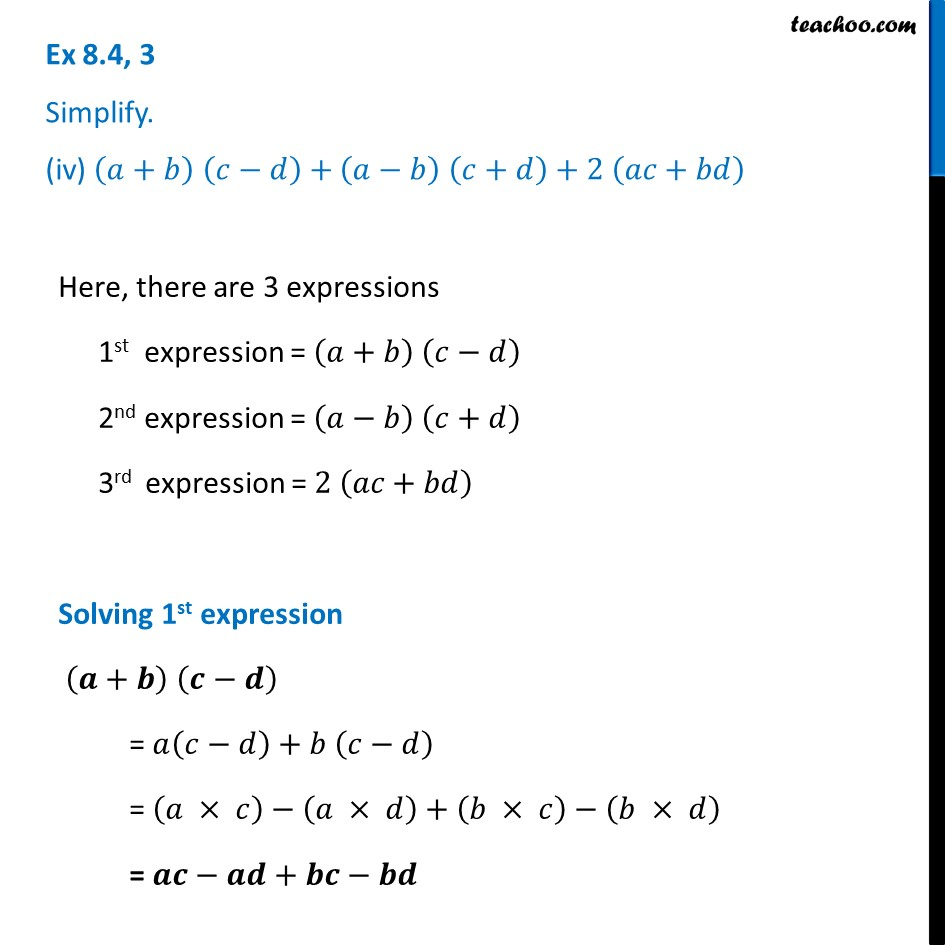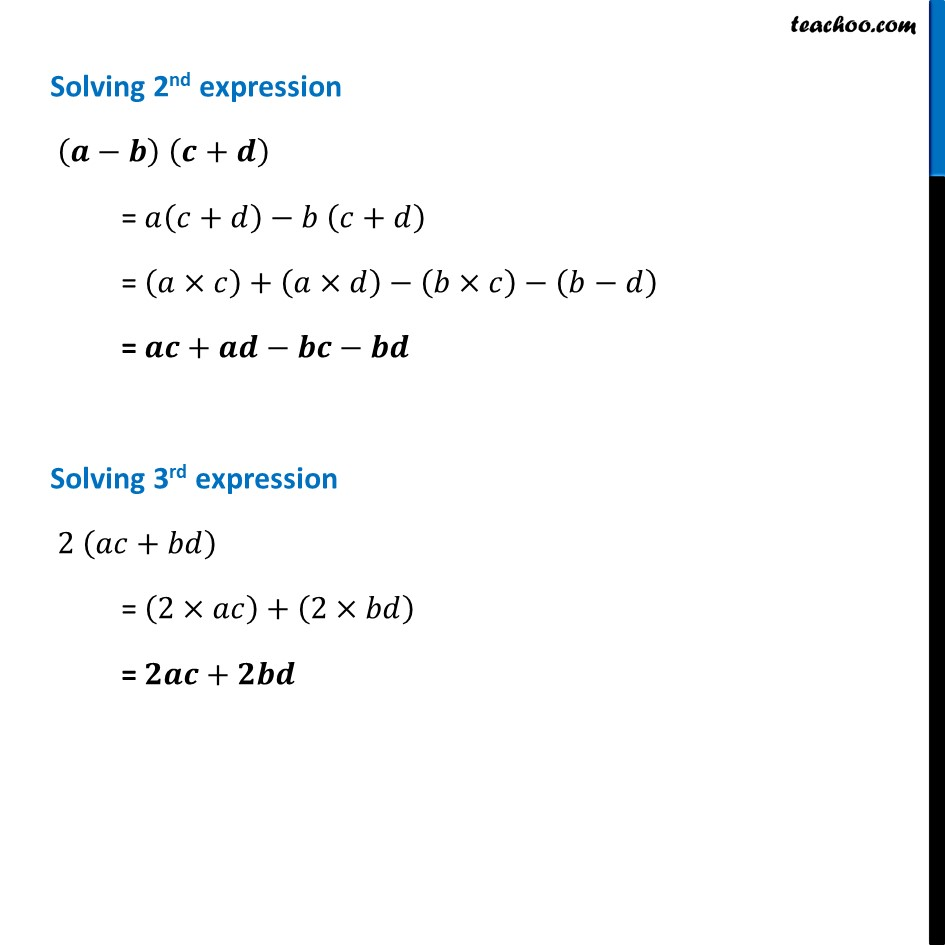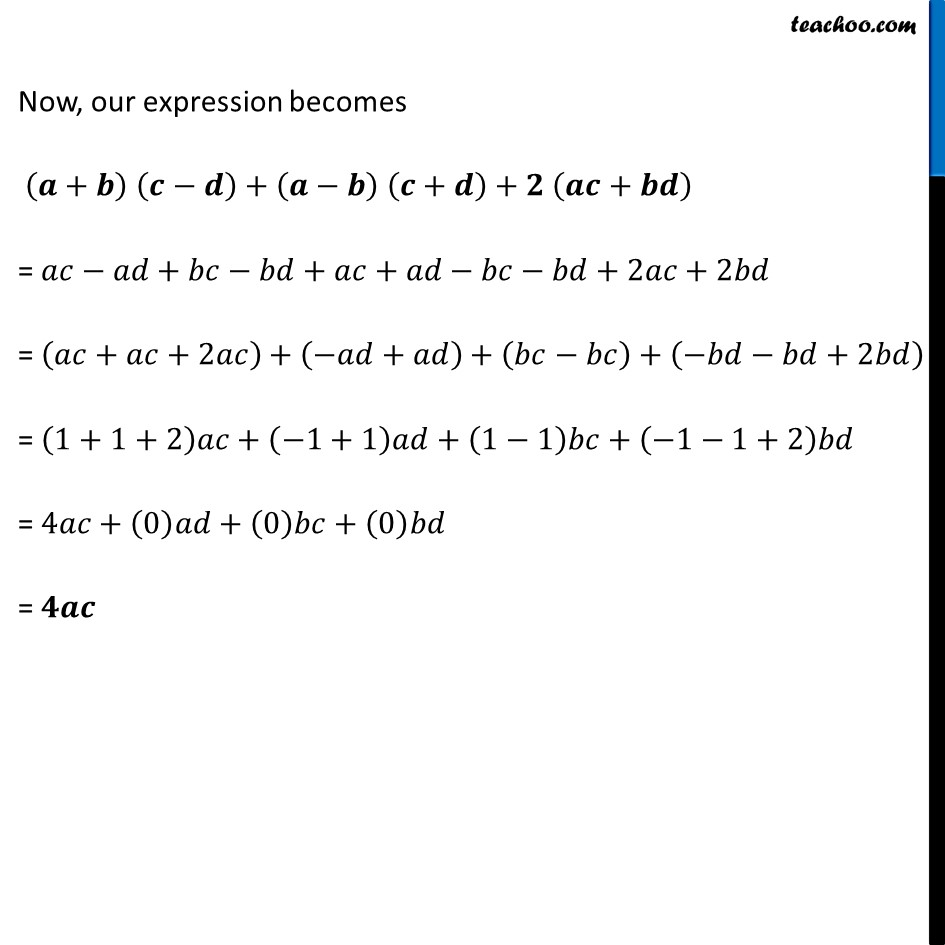Ex 8.4

Chapter 8 Class 8 Algebraic Expressions and Identities
Serial order wiseLearn in your speed, with individual attention - Teachoo Maths 1-on-1 Class

### Transcript

Ex 8.4, 3 Simplify. (iv) (𝑎+𝑏) (𝑐−𝑑)+(𝑎−𝑏) (𝑐+𝑑)+2 (𝑎𝑐+𝑏𝑑)Here, there are 3 expressions 1st expression = (𝑎+𝑏) (𝑐−𝑑) 2nd expression = (𝑎−𝑏) (𝑐+𝑑) 3rd expression = 2 (𝑎𝑐+𝑏𝑑) Solving 1st expression (𝒂+𝒃) (𝒄−𝒅) = 𝑎(𝑐−𝑑)+𝑏 (𝑐−𝑑) = (𝑎 × 𝑐)−(𝑎 × 𝑑)+(𝑏 × 𝑐)−(𝑏 × 𝑑) = 𝒂𝒄−𝒂𝒅+𝒃𝒄−𝒃𝒅 Solving 2nd expression (𝒂−𝒃) (𝒄+𝒅) = 𝑎(𝑐+𝑑)−𝑏 (𝑐+𝑑) = (𝑎×𝑐)+(𝑎×𝑑)−(𝑏×𝑐)−(𝑏−𝑑) = 𝒂𝒄+𝒂𝒅−𝒃𝒄−𝒃𝒅 Solving 3rd expression 2 (𝑎𝑐+𝑏𝑑) = (2×𝑎𝑐)+(2×𝑏𝑑) = 𝟐𝒂𝒄+𝟐𝒃𝒅 Now, our expression becomes (𝒂+𝒃) (𝒄−𝒅)+(𝒂−𝒃) (𝒄+𝒅)+𝟐 (𝒂𝒄+𝒃𝒅) = 𝑎𝑐−𝑎𝑑+𝑏𝑐−𝑏𝑑+𝑎𝑐+𝑎𝑑−𝑏𝑐−𝑏𝑑+2𝑎𝑐+2𝑏𝑑 = (𝑎𝑐+𝑎𝑐+2𝑎𝑐)+(−𝑎𝑑+𝑎𝑑)+(𝑏𝑐−𝑏𝑐)+(−𝑏𝑑−𝑏𝑑+2𝑏𝑑) = (1+1+2)𝑎𝑐+(−1+1)𝑎𝑑+(1−1)𝑏𝑐+(−1−1+2)𝑏𝑑 = 4𝑎𝑐+(0)𝑎𝑑+(0)𝑏𝑐+(0)𝑏𝑑 = 𝟒𝒂𝒄